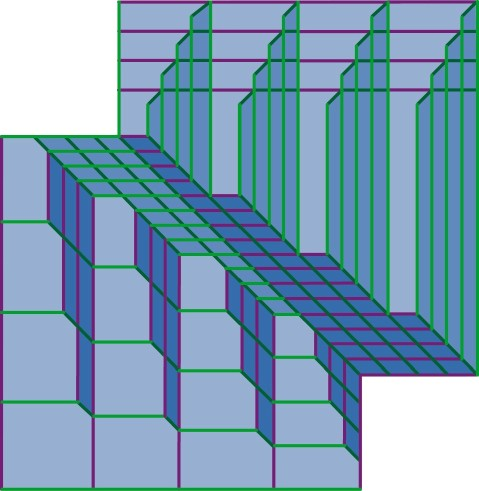Some duality conjectures for finite graphs and their group theoretic consequences Steve M. Gersten and Tim R. Riley Proc. Edin. Math. Soc., 48(2), pages 389–421, June 2005 We pose some graph theoretic conjectures about duality and the diameter of maximal trees in planar graphs, and we describe innovations in the following two topics in Geometric Group Theory, where the conjectures have applications. (i.) Central Extensions. We describe what we call an electrostatic model that constrains how the geometry of van Kampen diagrams change when one takes a central extension of a group. If the conjectures hold then, in particular, this theory gives a new proof that finitely generated class c nilpotent groups admit degree c polynomial isoperimetric functions. (ii.) Filling functions. We collate and extend results about how filling functions for finite group presentations interrelate. We use the electrostatic model in proving that the gallery length filling function, which measures the diameter of the duals of diagrams, is qualitatively the same as a filling function DlogA, which concerns the sum of the diameter with the logarithm of the area of a diagram. We show that the conjectures imply that the space-complexity filling function filling length essentially equates to gallery length. We give linear upper bounds on these functions for a number of classes of groups including fundamental groups of compact geometrizable 3-manifolds, certain graphs of groups, and almost convex groups. Also we define restricted filling functions which constrain diagrams with uniformly bounded vertex valence, and we show that, assuming the conjectures, they reduce to just two filling functions — the analogues of non-deterministic space and time. This article is the second in what was intended to be a series of three; the first is here. A proof of the conjectures was intended to be the subject of the third. They were resolved negatively in November 2005 — details are here.